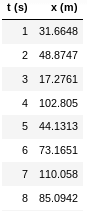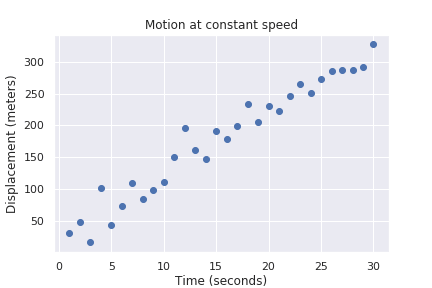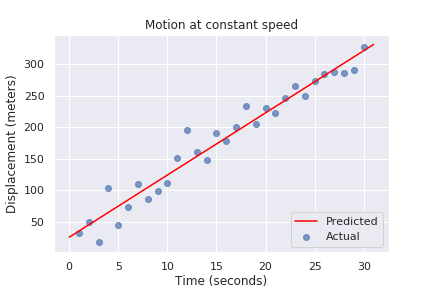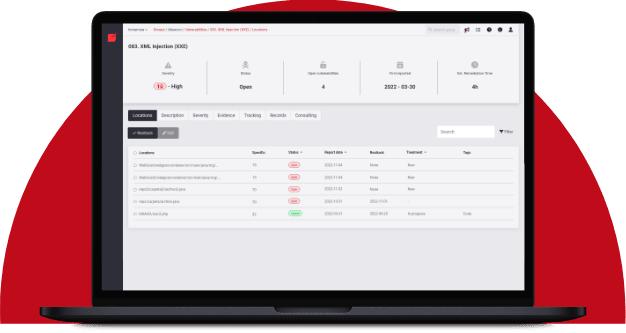# Digression to Regression

## Simple linear regression in scikitRafael Ballestas

After our risk quantification series, it should come as no surprise that we like numbers and measuring. However, when confronted with the question, "Why do you need to translate code into vectors?" I’m not sure what to say.

Almost all machine learning algorithms in abstract, and the concrete tools and libraries from the `Python` data science toolset (`Numpy`, `Pandas`, `Keras`) expect the features and targets to be real-valued. They rely on concepts such as distance, error, and cost functions, and they attempt to minimize the latter by adjusting their inner parameters. All of these concepts are inherently mathematical.

Out of all of these algorithms, the easiest to understand well is perhaps the simple linear regression. So, let’s start with that. Regression is a fundamentally different kind of task from the ones we have discussed the most in the series, which are classification and clustering tasks. Regression aims to predict a number taken from a continuum instead of answering "yes" or "no" or to tell from which group an input most likely belongs.

The situation is this: we have two variables that appear to be linearly related, and we would like a model that generalizes that relationship. Simple linear regression is used for many interesting purposes, such as determining:

• he relationship between the sales price of an asset vs. its age, i.e., understanding depreciation

• the fuel efficiency of a vehicle: fuel consumption vs. distance covered

• indirectly measuring physical quantities, such as speed or acceleration

In the first three examples above, it could be argued that there are other variables at play, that the relationship might not be necessarily linear, etc. But in the last case, and under lab-controlled conditions, it is true that the position x of an object that moves at constant speed v after a time t departing from an initial position i is given by x = vt + i. Suppose we want to know the speed v using only a ruler and a watch. If measurements were perfect, we would only need two, for we have two unknowns: v and i. However, in reality, measurements are not perfect, so we need to take several measurements, and sort of "average them out" via fitting a line, i.e., the regression. We can take several measurements of x and t, which we might register in a table like this:Figure 1. Position vs. time, first few observations

This doesn’t say as much as a plot:Figure 2. This shows positive correlation

Here we see there is a clear linear relationship, but no single line would perfectly fit all points. The goal in linear regression is to find the line that best fits the points. But what is the "best" fit? There are several choices, and each of those choices would represent a different model. The standard in linear regression is to minimize the sum of the squares of the errors for each observation. What? Each choice of v and i would produce, for each value of t, a corresponding value for x, which may be close or far from the actual observation. The difference between these two is called a residual. But we don’t care about each individual residual, we want only to make them small overall. So we add them all, but before that, we square them in order to avoid the possibility that due to the signs, they might end up adding to something close to `0`. This is, in fact, the cost function for this particular algorithm: different techniques come with different cost functions and different ways to minimize them.

## Get started with Fluid Attacks' Secure Code Review solution right now

In simple linear regression, the expression for the cost function is easy enough to solve with pen and paper. In other algorithms, hill-climbing and gradient descent techniques are taken from optimization. Even simple brute force (trial and error) might be used, as long as the cost function is minimized.

Instead of implementing simple linear regression in pure `Python`, which could be done, let this serve as an excuse to present the general machine learning flow. The tool of choice to perform "traditional" machine learning and data analysis in Python, i.e. everything but neural networks, will be scikit-learn. The flow to use this library is typically the same, regardless of how sophisticated the chosen model:

• Create an illustration of the model:

Creating a linear regressor in scikit.

``````from sklearn.linear_model import LinearRegression
model = LinearRegression(fit_intercept=True)
``````
• Train (fit) with the data
``````model.fit(t, x)
``````

Assuming that t and x are `NumPy` arrays (a more mathematically appropriate extension of lists) holding the time and position variables, respectively.

• Make predictions
``````import numpy as np
time = np.linspace(0, 31, 1000).reshape(-1,1)
xfit = model.predict(time)
``````

Create a new array holding a thousand evenly spread values for time, and then use the trained model to predict their corresponding values in the independent variable.

• Assess model performance
``````import matplotlib.pyplot as plt
plt.scatter(t, x, alpha=0.7)
plt.plot(time, xfit, 'red')
``````Figure 3. Regression line fits the data relatively well

In the case of simple linear regression, it is possible to see how well the model performed (see above). Quantitatively, we can use the R2 metric:

``````>>> model.score(t, x)
0.9531426066695182
``````

The closer this value is to 1, the better the fit, and hence, the regression.

That is, in a nutshell, how to make, train and draw predictions from machine learning algorithms in `scikit`, with a few subtle changes from one to the next, such as:

• Usually, one splits the data into training, validation and testing sets. However, the expected format remains the same. The features or predictors (t above, but usually x) need to be an array of vectors, and the targets or labels (usually y) a simple list or `1D` array.

• The validation and testing are performed in different ways according to the task, since plotting them and getting a single evaluating number as above is not always feasible.

Perhaps this helps to explain why you need to translate code into vectors. More later, on why, and how to turn natural language and code into vectors so that they might be used by standard ML techniques.

Share

Tags:

## Recommended blog posts

You might be interested in the following related posts.Why so many are switching to RustA roadmap for developing and releasing secure softwareVulnerability scanning and pentesting for a safer webInjecting JS into one site is harmful, into all, lethalDifferences between these security testing approachesOur CLI is an approved AST tool to secure cloud appsA simple approach to try out in cybersecurity trainingOpen the door to security as a quality requirement

## Start your 21-day free trial

Discover the benefits of our Continuous Hacking solution, which hundreds of organizations are already enjoying.Hacking software for over 20 years

Fluid Attacks tests applications and other systems, covering all software development stages. Our team assists clients in quickly identifying and managing vulnerabilities to reduce the risk of incidents and deploy secure technology.